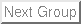Up: AARS Tutorial Previous: Exporting File Formats   Contents

Subsections

Appendices

Appendix A: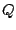The following equation is from the article Evaluationg protein structure-prediction schemes using energy landscape theory'' by Eastwood, et al.is the distance between a pair ofatoms.is the-distance between residuesandin the native state.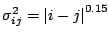is the standard deviation, determining the width of the Gaussian function.is the number of residues of the protein being considered.

Appendix B:The following text is in the article On the evolution of structure in aminoacyl-tRNA synthetases.'' by O'Donoghue et al.

Homology Measure

We employ a structural homology measure which is based on the structural similarity measure, Q, developed by Wolynes, Luthey-Schulten, and coworkers in the field of protein folding. Our adaptation of Q is referred to as, and the measure is designed to include the effects of the gaps on the aligned portion:=(+), whereis the normalization, specifically given below.is composed of two components.is identical in form to the unnormalized Q measure of Eastwood et al. and accounts for the structurally aligned regions. Theterm accounts for the structural deviations induced by insertions in each protein in an aligned pair: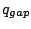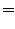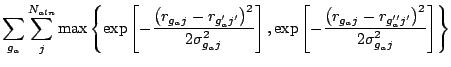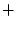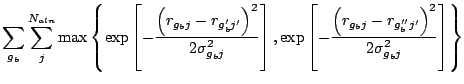The first term,, computes the unnormalized fraction of-pair distances that are the same or similar between two aligned structures.is the spatial-distance between residuesandin protein a, andis the-distance between residues' and' in protein b. This term is restricted to aligned positions, e.g., whereis aligned to' andis aligned to'. The remaining terms account for the residues in gaps.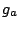andare the residues in insertions in both proteins, respectively.and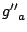are the aligned residues on either side of the insertion in protein a. The definition is analogous forand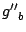.
The normalization and the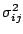terms are computed as: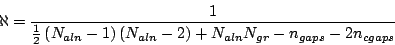where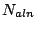is the number of aligned residues.is the number of residues appearing in gaps, and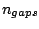is sum of the number of insertions in protein a'', the number of insertions in protein b'' and the number of simultaneous insertions (referred to as bulges or c-gaps).is the number of c-gaps. Gap-to-gap contacts and intra-gap contacts do not enter into the computation, and terminal gaps are also ignored.is a slowly growing function of sequence separation of residues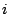and, and this serves to stretch the spatial tolerance of similar contacts at larger sequence separations.ranges from 0 to 1 where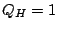refers to identical proteins. If there are no gaps in the alignment, thenbecomes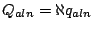, which is identical to the Q-measure described into themeasure described before.Up: AARS Tutorial Previous: Exporting File Formats   Contents
workshop+urbana@ks.uiuc.edu